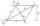# Pythagorean theorem - examples - page 4

1. InfinityIn a square with side 18 is inscribed circle, in circle is inscribed next square, again circle and so on to infinity. Calculate the sum of area of all these squares.
2. Rectangle SSPerimeter of a rectangle is 268 cm and its diagonal is 99.3 cm. Determine the dimensions of the rectangle.
3. LadderLadder 8 m long is leaning against the wall. It foot is 1 m away from the wall. In which height ladder touch the wall?
4. R TrapeziumRectangular trapezium has bases 12 and 5 and area 84 cm2. What is its perimeter?
5. TriangleCan be rectangular triangle equilateral?
6. OctagonWe have a square with side 84 cm. We cut the corners to make his octagon. What will be the side of the octagon?
7. Pyramid roof1/3 of area of ​​the roof shaped regular tetrahedral pyramid with base edge 9 m and height of 4 m is already covered with roofing. How many square meters still needs to be covered?
8. CapJesters hat is shaped a rotating cone. Calculate how much paper is needed to the cap 60 cm high when head circumference is 52 cm.
9. Cube - anglesCalculate angle between the wall diagonal and cube base. Calculate the angle between the cube body diagonal and cube base.
10. Sphere slicesCalculate volume and surface of a sphere, if the radii of parallel cuts r1=31 cm, r2=92 cm and its distance v=25 cm.
11. Tetrahedral pyramidCalculate the volume and surface area of a regular tetrahedral pyramid, its height is \$b cm and the length of the edges of the base is 6 cm.
12. RhombusFind the length of the other diagonal and area of rhombus. The perimeter of a rhombus is 40 cm and one of the diagonals is of length 10 cm.
13. ClimbOn the road sign, which informs the climb is 8.7%. Car goes 5 km along this road. What is the height difference that car went?
14. ISO triangleCalculate the area of an isosceles triangle KLM if the length of its sides are in the ratio k:l:m = 4:4:3 and has perimeter 377 mm.
15. Triangular prismPlane passing through the edge AB and the center of segmet CC' of regular triangular prism ABCA'B'C', has angle with base 22 degrees, |AB| = 6 cm. Calculate the volume of the prism.
16. Recursion squaresIn the square ABCD is inscribed a square so that its vertices lie at the centers of the sides of the square ABCD.The procedure of inscribing square is repeated this way. Side length of square ABCD is a = 22 cm. Calculate: a) the sum of perimeters of all
17. Diagonals of the rhombusCalculate height of rhombus whose diagonals are 12 cm and 19 cm.
18. Rhombus 2Calculate the area of rhombus which has a height v=48 mm and shorter diagonal u = 60 mm long.
19. Leg and heightSolve right triangle with height v = 9.6 m and shorter cathetus b = 17.3 m.
20. Similarity coefficientThe ratio of similarity of two equilateral triangles is 3.5 (ie 7:2). The length of the side of smaller triangle is 2.4 cm. Calculate the perimeter and area of ​​the larger triangle.

Do you have an interesting mathematical example that you can't solve it? Enter it, and we can try to solve it.

To this e-mail address, we will reply solution; solved examples are also published here. Please enter e-mail correctly and check whether you don't have a full mailbox.

Pythagorean theorem is the base for the right triangle calculator.